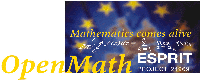Next: Conclusions Up: The OpenMath Project Final Previous: Software Tools   Contents

Subsections

# Mathematical Applications

In this section we describe the work done in the project building mathematical applications using OpenMath. There were two main approaches used: to add OpenMath support to an existing application, or to construct a new application using OpenMath tools.

## Writing Phrasebooks for Existing Mathematical Software

The reader will recall that the layer of software which translates between an OpenMath encoding and a piece of software's own internal format is called a phrasebook. The task of adding OpenMath support to an existing application is therefore mainly the process of writing one or more phrasebooks, although it can also involve the specification of new content dictionaries. More than one phrasebook might be required if an application is expected to interpret the same input in different ways, for example a computer algebra system would generally simplify the OpenMath expression:


<OMOBJ>
<OMA>
<OMS cd="transc1" name="arcsin"/>
<OMI> 1 </OMI>
</OMA>
</OMOBJ>

to something like:

<OMOBJ>
<OMA>
<OMS cd="arith1" name="divide"/>
<OMS cd="nums1" name="pi"/>
<OMI> 2 </OMI>
</OMA>
</OMOBJ>

(i.e.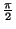, although+ 2 n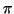, n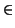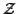would be better). However, in some circumstances it might be required to do computations in floating point, in which case it might return

<OMOBJ>
<OMF hex="182d4454fb210940"/>
</OMOBJ>


Writing a phrasebook requires a great deal of knowledge about the semantics of the underlying application and expertise in this area is more important than expertise in OpenMath (since its semantics are very explicit). The project has developed a number of libraries (see 6.1) to assist in the reading and writing of encoded OpenMath objects. In addition there have been some experiments with generic phrasebooks designed to carry out the translation as a separate process.

## AXIOM

AXIOM is a strongly-typed computer algebra system marketed and developed by NAG Ltd. The system is implemented in its own special-purpose language which runs on top of a Lisp kernel. During the project NAG experimented with adding OpenMath support to the kernel by building on top of the C library developed by INRIA (see 6.1), extending some of AXIOM's data types so that they could export instances of themselves using the XML encoding, and adding the capability to run as a server, reading and writing OpenMath objects on a socket. Some of these features will be included in the next commercial release of AXIOM (AXIOM 2.3), in late 2000.

## Reduce

Early in the project a student from the University of Bath implemented a MathML package for Reduce, which extended the system to accept input and print results in that format. NAG built a wrapper for Reduce which used an XSL transformation engine to convert OpenMath to and from MathML so that it could operate as a simple server. While slow, this was a quick and re-usable solution which provided an interesting test bed for both the XSL stylesheets (see 6.5) and the Reduce MathML support.

## The Multiple Integrators Demonstrator

One advantage of OpenMath is that it can lead to a plug and play'' approach to linking together mathematical components. To demonstrate this a Java applet was developed which used STARS (see 6.3.1) as an editor/rendering tool which allowed a user to enter an expression to be integrated. The formal integral was shipped to either the AXIOM or Reduce servers (as described above) to be evaluated. The resulting expression was displayed in the applet using a second instance of STARS.

## GAP

GAP is a system for computational discrete algebra developed by a worldwide community and currently maintained by the University of St Andrews. It is distributed under copyleft'' conditions, i.e. it can be used freely by anybody but not included in a commercial product.

A GAP share package was developed at St. Andrews and is now available to users via FTP. It uses an application based on the INRIA C Library (see 6.1) to read and write OpenMath objects from files, sockets etc.

## Mathematica

A phrasebook for Mathematica was developed at INRIA, based on the MathLink protocol and the OpenMath C Library (see 6.1). Its design is similar to that of the GAP phrasebook in that it is a separate process which sits between Mathematica and the client application, although the implementation details are different. It can be obtained by contacting the INRIA team.

# The NAG Library

NAG is perhaps best known for its numerical libraries, which are written in a variety of languages including Fortran-77. An important facet of these libraries is the comprehensive nature of the documentation which describes the background to each algorithm, the parameters of each routine, details of how to interpret errors and a fully-worked example. As well as printed documentation, NAG has used a number of online formats over the years, with PDF being the current favourite (because of its cross-platform nature and ability to render mathematics faithfully). As an experiment NAG decided to produce a version of one of its routine documents which used MathML to display mathematical expressions, and provided interactive access to the routine using OpenMath.

The approach chosen was to allow the user to input the problem (in this case constrained minimisation of a multivariate expression) in a Java applet running inside an appropriate (in principle MathML-supporting) browser. The problem would be encoded as OpenMath and sent to a server which was capable of executing the NAG routine and returning the result. Unlike the previous examples, this server would be written from scratch as an OpenMath application. Its interface would be defined in terms of an OpenMath object described in a private content dictionary (although the individual components were defined using symbols from standard CDs).

This server can be used in a variety of contexts provided that the input is encoded in OpenMath (as opposed to binary data laid out in memory according to ANSI Fortran rules, for example). Equally, since the interface is well-defined, it would be possible to use a different server (incorporating a more powerful NAG routine or the Matlab optimisation toolbox or ...) from the client relatively easily.

The components were constructed using generic tools. The client was written in Java and made use of the STARS (see 6.3.1) applet from Stilo for translating the user input to OpenMath and displaying it in MathML. The server was written in Aldor  and used the Aldor OpenMath Library (see 6.1) for reading and writing the OpenMath objects. It also used standard Aldor bindings to the NAG Library to solve the problem. While we could have written everything from scratch the availability of all these tools made building the software much more straightforward.

This experiment has proved extremely successful and NAG is now considering the development of a toolkit to allow the rapid deployment of its algorithmic software in this way.

# Electronic Journal Databases

The London Mathematical Society's Journal of Computation and Mathematics is an electronic-only subscription journal, published in PDF since 1988. The advantages of being an electronic-only journal are cited in the editorial as follows.
• Speed.
• Flexibility.
• Updating.
• Searchability.
It is this last that the OpenMath Project wished to address. Although the Journal is published in PDF, the sources of all the papers, in LaTeX, are maintained by the Journal7.1. The Journal does have a full-text search capability, driven off a database built from the LaTeX sources.

This is far from perfect, since there is much information in the formulae that cannot be recovered from the text. A few examples of this are given below.

1. A search for the word dihedral'' reveals  and . However, a search for the word Frattini'' only reveals , even though Frattini groups are mentioned in , but only by their symbol.
2. Some terms may be multiple words, which are harder to find. An example is the phrase general linear group'', which is traditionally represented7.2 by the mathematical symbol GL.
3. There may be more specifics in the notation. For example,  contains the phrase as an application of this result, we work out the case GL4(q)''. If one was only interested in details of GL4, a text-based search would be less use than a pattern-matching formula-based search on GL4(*) - in fact, due to the nature of MFD2's unification engine, a search on GL(4, x) for any OpenMath variable (OMV) x would recover this formula: there is no need to know that it is q.

We therefore built a prototype formula search engine on some of the formulae in the abstracts of the LMS JCM. As envisaged in the plan, this was built on top of the MFD2 search engine  from INRIA (see section 6.2). The formula search engine was given a database of formulae from the abstracts of the journal (there is no reason why this could not be from the whole journal, but for the purpose of this exercise it was felt that concentrating on the abstracts of as many articles as possible was better than looking at a few articles in depth). While time did not permit a user-driven evaluation, the points described in the following sub-sections were noted.

## OpenMath points

The following points relate largely to the details of OpenMath, its use, and the CD mechanism.
• None of the four occurrences ofin the abstracts mean 3.1415926...: all refer to various projections. Of course, this is easy in OpenMath7.3, rather than being

<OMS cd="nums1" name="pi"/>

they would be

<OMV name="pi"/>

or

<OMS cd="..." name="pi"/>


• Many of the ideas present were not in existing CDs. For the purpose of that task, some ad hoc CDs were written, but this did also prompt the investigation into an asymptotics CD, which turned out to raise several interesting questions (see section 7.3.4).

## JCM points

The following points relate largely to the JCM and the LaTeX in it.
• There is no consistency of presentation - as remarked above, it could be GLn(K) or GL(n, K). Whether there should be is dubious: there are differences in presentation between sub-disciplines (consider the i versus j for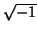'' battle), but the question needs to be asked, and the OpenMath discipline helps.
• Even after the attention of an extremely careful copy-editor, the quality of the LaTeX is poor for semantic purposes. Consider the following extract from  (the wider context is quoted below in section 7.3.3):

$O(\ell N^2 \log N$)

Clearly what is meant is the transposition of the last two characters:

$O(\ell N^2 \log N)$

which prints so similarly that the difference cannot be detected by examining the paper result.

Unfortunately for any hopes of automatic correction, the formula given is inside parentheses, so the misplaced ) would be picked up as closing the parenthesis. One cannot require that a LaTeX formula be parenthesis correct'', because of interval notations such as [0, 1) (or the variant [0, 1[ common in France).

## Text, data or both?

Overall the separation between text and data is not very helpful. A classic example is given by , which says the following.
An algorithm is given that recognises (in O(lN2log N) time, where N is the size of the input and l the depth of a precalculated Schreier tree) when a transitive group (G,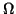) is the action on one orbit of the action of G on the set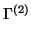of ordered pairs of distinct elements of some G-set (that is,is isomorphic to an orbital of (G,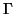)).
Here the relation between the formulae (e.g. N and G), which would be vital for any decent understanding of the mathematics, is carried by the text. Techniques such as OMDoc [2,3], which build on OpenMath, may well be necessary to make any sense of this.

The situation is even worse if we consider the abstract of .

We give an algorithm that takes as input a transitive permutation group (G,) of degree n = mchoose2 and decides whether or notis G-isomorphic to the action of G on the set of unordered pairs of some seton which G acts 2-homogeneously. The algorithm is constructive: if a suitable action exists then one such will be found, together with a suitable isomorphism. We give a deterministic O(snlogcn) implementation of the algorithm that assumes advance knowledge of the suborbits of (G,). This leads to deterministic O(I2) and Monte-Carlo O(snlogcn) implementations that do not make this assumption.
Here I is totally undefined (one guesses that it is the size of the input), as are c and s.

## Implicit mathematical information

In general, much mathematics is implicit. This is, we found, particularly true of the O notation and related ones. In the quotation from  above, consider the formula O(lN2log N). This is clearly'' intended to be (apart from the influence of l)

 ON - >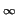(lN2log N). (7.1)

However, in the formula

sin(k2x) = k2x - (k6x3)/6 + (k10x5)/120 + O(k14x7),

the O expression is, equally clearly'', intended to be

 Ox - > 0(k14x7). (7.2)

How should OpenMath express the extra information in equations 7.1 and 7.2? It would have been possible to base the syntax very closely on that of the limit symbol. This would, in some sense, reflect the underlying formal mathematics, but probably not the way these symbols are used in practice.

Instead, using the same methodology as in diff and int it was decided to make the main argument of O into a lambda-expression. While this might seem to cause problems on the rendering side (who would want to see O(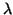x.x7)?), these problems have already been solved for diff and int. One advantage of this is that it is possible to deal with functions of more than one argument, as in the translation of equation 7.1, which would be structured as follows (ignoring the complexities of causing the l to render as l, which are not germane to this discussion).


<OMA>
<OMS name="O" cd="asymp1"/>
<OMBIND>
<OMS name="lambda" cd="fns1"/>
<BVAR>
<OMV name="N"/>
<OMV name="l"/>
</BVAR>
<OMA>
<OMS name="times" cd="arith1"/>
...
</OMA>
</OMBIND>
<OMA>
<OMS name="list" cd="list1"/>
<OMS name="infinity" cd="nums1"/>
<OMS name="infinity" cd="nums1"/>
</OMA>
</OMA>


It was decided to make the place to which the implicit limit is taken be a second argument to the O symbol. This allows for a value of unknown in places where the OpenMath generator is unable to determine a sensible value.

## Conclusions

As a proof of concept'', which is all it was intended to be, this task has been a success. It has demonstrated that it is possible to build a database of OpenMath formulae from real-life examples, which can be intelligently searched by a suitable search engine. It should be noted that MFD2, the search engine used, has the sophisticated unification capabilities that are necessary for this task - one man's k is another man's l''.

However, many areas for future development have been highlighted by this application of OpenMath to real life'' data.

1. The poor quality'' (in terms of semantic content) of the LaTeX available - it is a presentation language, and people judge its output as such: see the second item in section 7.3.2.
2. The difficulty of LaTeX -> OpenMath. Based on the evidence gathered in this task, this is the subject of an application to the U.K. research councils (EPSRC). A key point here is that such translation needs to be domain-specific within mathematics: in the JCM's current contents nowas 3.14159..., but other papers could have changed this easily.
3. The requirement for Content Dictionaries. While some of these are fairly easy to write (e.g. one for GL), others are by no means as easy to write as one would think - see section 7.3.4.
4. The difficulty of divorcing the semantics of formulae from those of the text, and the inter-relation between the two. The data from this task lend weight to the need for a unified approach [2,3].

# Electronic Books

In order to experiment with OpenMath in the creation of electronic books, the interactive book Algebra Interactive!'' has been produced. The first version used JavaScript, Java applets and GAP. It was set up so as to be able to implement OpenMath, although OpenMath itself was not yet present. It has been released as a commercial product by Springer Verlag .

The book is an introduction to abstract algebra for first year undergraduate students in Mathematics, Computer Science and Electrical Engineering. It distinguishes itself contentwise by its focus on algorithms and applications. Interactivity is obtained through

• interesting playful applets, that require little knowledge of the content, but try to interest the student in the topic;
• three kind of interactive exercises: first, a list of `regular exercises' with hints and solutions obtainable on request, second, a challenge to see if a theorem is well understood by means of a single multiple choice question attached to the theorem, and third, a multiple choice test based on random selections from a data base for each chapter, enabling the students to test their advancements;
• so-called gapplets: about 130 screens with linear input facilities and linear output, where GAP is used as a back engine to provide the student with less trivial examples than can be computed by hand in a short time span;
• the usual links and indices for an electronic book.

The authors of Algebra Interactive are Arjeh M. Cohen, Hans Cuypers, and Hans Sterk. Many core Java applets have been written by L. van Gastel, A. Heck and G. Simons. Technical assistance was carried out by C. Huiban and W. Kortsmit. At Springer-Verlag, details regarding design, layout, software, and commercial aspects were sorted out by A. Einzmann, M. Feith, T. Fuhrmann, F. Schmidt, V. Wicks. Further contributions at RIACA/EUT came from S. Ball, A.E. Brouwer, A. Blokhuis, J. Geraats, W. de Graaf, S. Hoop, M. Lavrauw, R. Lindenbergh, S. van Rijnswou, M. Smeets, A. Steinbach, R. Ushirobira, J. Veerman, R. Verstappen, and H.A. Wilbrink. The GAP development team in St. Andrews helped including GAP as a back engine in Algebra Interactive and provided useful suggestions. The integral source and running version of GAP are to be found on the CD Rom.

The idea at the last stage of this subproject was to translate the content files of the electronic version of this book, usually referred to as IDA1 within the project, in such a way that the mathematics would be encoded in OpenMath. Joint study with Michael Kohlhase en his group at Saarbrücken has led to the definition of an XML standard for such a document, called OMDoc. The reason behind this choice of a new standard is that more than simply OpenMath is required to set up the structure of an interactive book (or document). An important OMDoc feature is that both the CD's (conceptually) created within the text and the OpenMath object present in the document can be extracted automatically. By means of ad hoc programming, a first translation from the IDA1 source texts to OMDoc has been achieved. Furthermore, Chapter 1 of IDA has been manually reworked to a full OMDoc. Using the latest Mozilla browser (and its MathML display capabilities), this chapter can be professionally visualized. All mathematics, including the symbols inserted in sentences, are in OpenMath, as can be visualized by clicking them (forcing the raw OpenMath to appear in a popup window). This shows that the expressions are "ready for use" in any conceivable application. Further progress on interfaces with backengines has given us the possibility to formulate in the OpenMath language the queries needed for interactivity of the gapplet kind (as enabled in IDA1). This OpenMath version of the gapplets appearing in OMDoc are called "omlets". A direct positive effect is that the output of omlets now appears naturally displayed in the text; this is a huge improvement over the clumsy ASCII string displayed in IDA1 as gapplet output. The big improvement however is that we now have a very flexible source, from which many versions of the book (and personally adapted displays) can be made by means of style files.

The new version has been shown at the International Conference in Lisbon, November, 2001, by Dr. Hans Cuypers of RIACA/EUT Eindhoven.

SMASH, Springer's Mathematical Assistant System Heidelberg is based on the technology for IDA2. The goal is to create a collection of pages containing useful concise information for the engineer for each relevant topic, with back engines enhancing the interactivity, and possibly data bases supporting the knowledge supply. The use of the backengines GAP and Mathematica (via JLink) has been successfully demonstrated. A convincing prototype on prime numbers has been completed, and the outlook for a major project in this direction is promising. The idea would be that a future version or successor to JOME will play the role of an authoring tool, and that database tools, extending MDF2, will be used in which OpenMath helps to find the expressions in the database corresponding in a meaningful way to a given query.

#### Footnotes

... Journal7.1
Another reason for this is future-proofing: when new formats become common, and PDF and LaTeX die out, it seems likely that it will be much easier to roll the LaTeX forward to the new formats than the PDF.
... represented7.2
This is actually a good example of power of OpenMath, since in fact there are two presentation notations in common use: GLn(K) and GL(n, K). However, in OpenMath, both would be represented by the same semantic notation, and the choice of presentation would be up to the OpenMath -> MathML-P converter.
... OpenMath7.3
But much harder for the LaTeX -> OpenMath translator, and indeed this was one of the areas where manual intervention was required.Next: Conclusions Up: The OpenMath Project Final Previous: Software Tools   Contents
The OpenMath Consortium